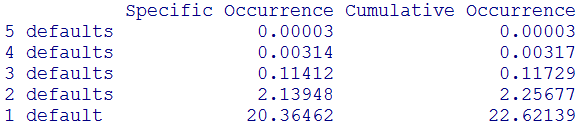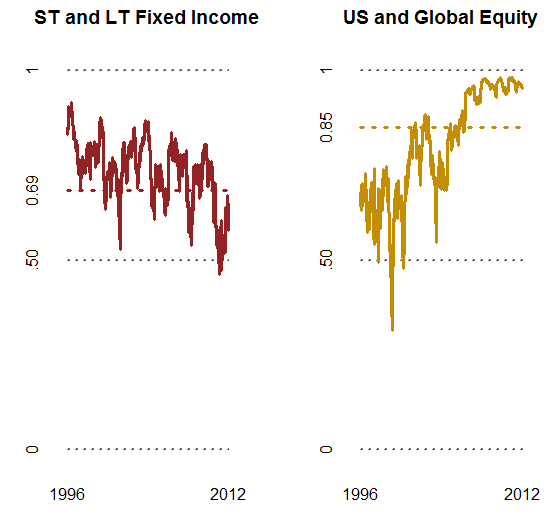## Thursday, June 5, 2014

### When Correlations Go To One

Recently a co-worker lent me Nate Silver's The Signal and the Noise. In the first chapter the author discussed the financial crisis and how mortgage pools change based on the correlation of their risks.

In prior posts (see Mei's Monte Carlo Adventure for example) we have modeled investment portfolios assuming that the correlation between securities was constant. We have always cautioned, however, that this assumption may not be correct.

In his book, Mr. Silver provided some data on how default rates in mortgage pools change under both an uncorrelated assumption and a perfect correlation assumption, and I thought it would be fun to replicate this and to take a deeper dive into what happens when correlations change.

The Uncorrelated Pool

Say we have bought 5 mortgages, and the risk of default on these is 5%. If this rate is too risky for some investors, we can create an investment that they can invest in by using them to create a different type of security.

We place the 5 mortgages together, creating a "pool". We then sell 5 securities to the market, A through E, whose payout will be based on order of default. Securities A through E are called "tranches" of the pool.

If all mortgages pay out, all 5 of our created securities pay investors. If one defaults,then investors in A through D get paid but E does not. If two default, investors in A through C get paid but D and E do not, and so on.

Figure A
Mortgage PoolsFive mortgages are pooled and five new securities are created. Payout for each security is based on the number of defaults.

Figure A depicts this structure.

One way to think about this is to imagine we have large soup pot on the stove. Each of the payments for the five mortgages are the ingredients that go into the soup.

After we stir a little bit, we ladle out the soup, only we do so in a certain order. Person A always gets the first ladle full, Person B the second, and so on. If one of the mortgages defaults, so we only have 4 ladles full of ingredients going into the soup, then there will not be enough for person E when they show up holding out their bowl.

Figure B
Uncorrelated Default RatesFirst column is occurrence of the specific event - answering the question 'what percent of the time was there exactly x defaults?'. The second column is cumulative occurrence, answering the question 'what percent of the time was there at least x defaults?'

If we assume that the mortgage defaults are uncorrelated - meaning that if one defaults it in no way indicates that any of the other four default, then the probabilities of default occurrence for each of our newly created securities will be close to that of the results in Figure B, which is R output for a simulation of 10 million runs. Sometimes we will hear the phrase 'the variables are independent', which is stat-speak for uncorrelated.

These results match (with rounding) those produced in Mr. Silver's book.

Back to our mortgage situation, we had the case where an investor would not be able to purchase the 5% default risk mortgage securities because of their risk.

By creating our pool structure, we have created mortgage securities where three of them (A, B and C) have less than 0.12% probability of default, a much less risky proposition for these investors.

Of course, these results only hold if the securities are in fact uncorrelated.

The Correlated Pool

In the prior example the mortgages going into the pot were uncorrelated, meaning that the behavior of one does not impact the other.

In our simulation every now and then a mortgage would default and person E did not get paid. In a few instances 2 mortgages defaulted at the same time, but the other ones did not. In well over 99% of the simulation cases no more than 2 defaulted at any one time, so persons A through C would almost always get paid.

However, if the mortgages are correlated, meaning they move more "together" rather than "independently", this dynamic changes.

At the extreme, if the correlation is 1 (the highest a correlation can go), then when one defaults all five of them default. This means either there is soup for everyone or soup for no one - nothing in between.

In this case, the benefit of carving out different tranches of the pool is of no benefit whatsoever. The investor may as well have invested in one mortgage with a 5% chance of default, because that is the same probability as their perfectly correlated tranche security offers.

Figure C
Bond Prices By TrancheBond prices are calculated based on (loss given default * probability of default)/risk-free rate

This brings us to an interesting point. By creating a structure based on one assumption, should that assumption change it creates both winners and losers. Figure C shows theoretical bond prices based on the probability of default and 100% loss under that scenario.

Under the uncorrelated scenario, tranche E is worth about 73.69 cents on the dollar, since it is the tranche that gets hit with the vast majority of defaults.

However, under the perfect correlation assumption it is worth a little over 90 cents on the dollar. All the other tranches experience decreases in value from the uncorrelated to perfectly correlated scenarios.

In total, however, the entire pool of bonds is worth the same amount. This has to be so, as we have not changed the inherent risk of the mortgages (i.e. 5% default probability) in either case, only the correlation.

The Spaces In Between

Between the perfectly correlated and the perfectly uncorrelated, we have the partially correlated.

In Cholesky to the Rescue, we discussed how to create partial correlation between different simulated events according to a target correlation matrix.

But in that situation, we were using the normal distribution. In the mortgage default case, we are using the uniform distribution, one that has a range between 0% and 100%. There is no normally distributed mean or standard deviation to use like there is in the former case.

How do we go about creating a simulation that creates correlation between variables while staying true to the original variables' uniform distribution parameters?

For one answer to this, we can turn to the work done by Enrico Schuman in his post Generating Correlated Uniform Variates.

In this approach, we perform 4 steps.

::Create our correlation matrix
::Convert the matrix to Spearman correlation
::Simulate variables using the Cholesky decomposition of this matrix using normal distribution
::Convert the normal variables to the uniform distribution by using their p-values (probability of occurrence)

Under this method, we can use the well-developed normal distribution processes and simply convert the results to the uniform distribution in a manner that maintains the correlation.

Figure D
Default Probability at Different Correlation LevelsAs correlation trends towards 1 default probabilities converge to .05

Figure D shows the default rates for each tranche of our pool resulting from one thousand simulations performed at each level of correlation (to the second decimal point, or for each .01). During each simulation the variables were run 100 times, so the total for each correlation level is 10,000.

As the correlation approaches 1, each of the security's default rates converge to 5%. The change in the price of these securities shown in Figure C, will change as the lines in Figure D change (opposite the sign of the change, so increases in Figure D mean decreases in bond prices).

The impact to each tranche varies. Tranche D's probability of default almost immediately starts to increase (thus it's value is decreasing) as things become more correlated, while Tranche A's holds out almost to the very end, but then increases rapidly.

Tranche D also has the interesting dynamic that it's probability of default increases and then decreases - the only security to do so. All the others only increase or only decrease.

What You Can Do

Correlation is a condition often stipulated for a model but not always completely thought out. Yet its' impacts in certain situations can be quite significant. Some questions to ask when analyzing our models or the results from them are:

What happens if our correlation assumption changes? - using Figure C as our example, asking this question allows us to consider the fact that our correlation assumption will drive a 5% change in our investments value.

Figure E
Average and 100-Day Rolling CorrelationAverage correlation for entire time period is shown by the colored dashed line, and the rolling 100 day correlation by the solid lines

How strong is our correlation assumption? - For whatever period of time we calculate a correlation, we are inherently assuming that it will apply to the time period going forward. This is almost never the case. Figure E shows the correlation between Short and Long Term Fixed Income funds and US and Global Equity Funds (original data from Should You Rebalance Your Investment Portfolio?) from 1996 to 2012. The entire period correlation is almost never equal to the 100 day correlations, and the 100 day correlations can change dramatically in relatively short periods of time. In addition, there are distinct correlation differences in different time periods, such as equity correlations being slightly higher than .5 (and volatile) for much of the 90's compared to being close to 1 during the past few years (and not so volatile).

Insist on Scenario Analysis - one way to achieve the objectives in our first two "What Can You Do" items, we can insist on reviewing results of scenarios where the correlation conditions have been altered. For example, looking at the Equity correlations in Figure E, some possible scenarios are "Maintain recent high correlation", "Regress to the average", "Intermittent periods between high and low", "Return to the 90's", and "Go to Zero". Comparing the results of these scenarios will help us to understand the risk potential, though not necessarily the probability of each.

Learn From the Traders - a book I read once (though the name of it escapes me) made the claim that in security trading "every trader's position will eventually get wiped out", implying that trading is fundamentally a race to cash out before that eventuality occurs. Long Term Capital Management did great...until it didn't. Mortgage Bond traders and banks did great...until they didn't. So it will go with correlation, at some point the uncorrelated will go to 1, or the strongly correlated will go to 0. We need to make sure we are clear what we will do when that occurs.

Key Takeaways

The assumptions that go into a model are essential to the results it generates. Investigating what can occur if a) the assumptions change, or b) are simply incorrect, is an essential component of building an actionable analytical framework.

You May Also Like
Questions
::What assumptions have you challenged that significantly impacted the results of a model's output?
::What is the best way to proceed if correlation is uncertain?
::Do you agree that eventually "all correlations will go to 1" or "all correlations will go to 0"? Why or why not?